# Principles of CD/ORD (1): Principles of Circular Dichroism and Optical Rotatory Dispersion

October 6, 2020

The electromagnetic nature of light is described in the 'Basics of UV-visible spectrophotometry (1) Properties of light'. Electromagnetic waves contain electrical and magnetic field components that oscillate perpendicularly to the direction of propagation of the light beam. In natural light, these components vibrate in many directions. On the other hand, in linearly polarized light, these vibrations are confined to a single plane (Fig. 1-1, upper). Another form of polarization is circularly polarized light, in which the vibration plane rotates as the light beam propagates (Fig. 1-1, lower).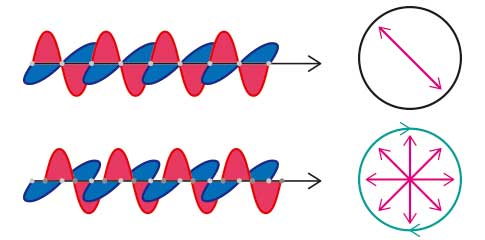Fig. 1-1 Linearly polarized light (upper), circularly polarized light (lower)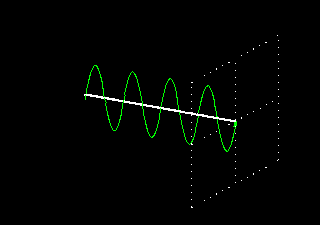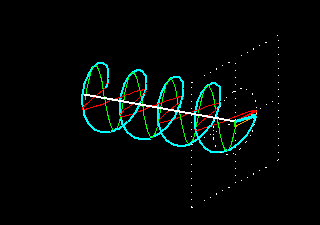Fig. 1-2 Linearly polarized light vs. circularly polarized light*

Linear polarization is obtained by passing light through an optical element (polarizer) in which the crystal axes and the orientation of the molecules are aligned. Circular polarization occurs when the phase of an orthogonal wave vector (electric field vector) deviates by 1/4 wavelength. Circularly polarized light can be left-handed (El) and right-handed (Er), and linearly polarized light can be treated as the sum of E l and E r of equal intensity (Fig. 2, upper). When E l and E r have different intensities, the resulting light is referred to as elliptically polarized (Fig. 2, lower).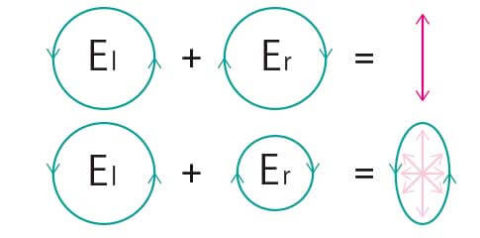Fig. 2-1 Sum of circularly polarized light components
El = Er： linearly polarized light (upper)
El ≠ Er：elliptically polarized light (lower)Fig. 2-2 Sum of circularly polarized light and linearly polarized light*

## Optical rotation and principle of ORD measurements

Optically active substances are molecules that have mirror images with L- and D-forms. Examples of such molecules are thalidomide and glyceraldehyde. Such substances cause a rotation of the polarization plane of linearly polarized light, referred to as optical rotation (OR). The wavelength dependence of OR is called optical rotational dispersion (ORD).
As shown in Fig. 3, when light that is linearly polarized along the Y-axis has its polarization plane rotated to the left, this is referred to as levo (l) or (-) optical rotation. When the rotation is to the right, it is referred to as dextro (d) or (+) optical rotation. The rotation angle is given by α, which can be positive or negative.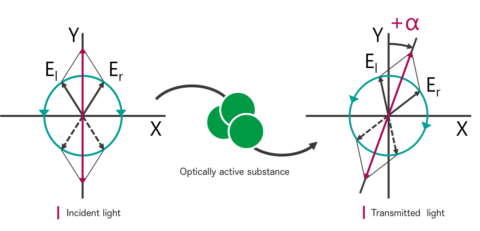Fig. 3 Principle of optical rotation

## Principles of CD measurement

Circular dichroism (CD) is a phenomenon in which the degree of absorption of left and right circularly polarized light is different in the absorption wavelength range of optically active substances. CD measurements detect these differences by passing left and right circularly polarized light through an optically active sample. The transmitted light is then elliptically polarized, and this phenomenon is called circular dichroism (CD) and is expressed by the ellipticity θ (Fig. 4). A plot of the wavelength dependence of this ellipticity is called a circular dichroism spectrum (CD spectrum).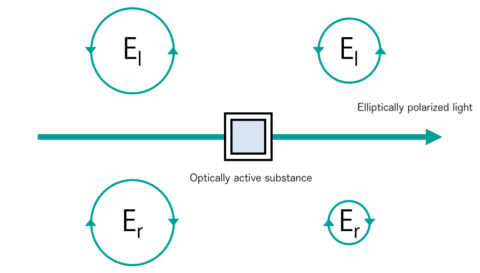Fig. 4-1 Principles of CD：unequal absorption of circularly polarized light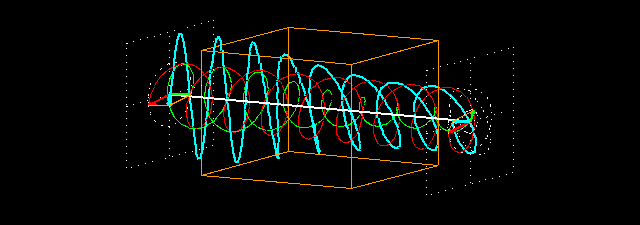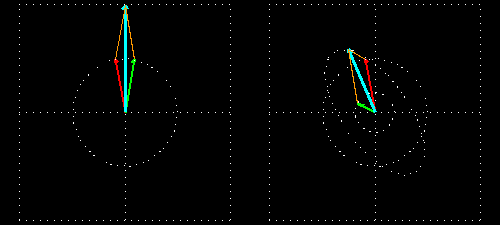Fig. 4-2 Principles of CD：Unequal absorption of circularly polarized light*

*EMANIM animations used with permission by Dr. Andras Szilagyi: http://emanim.szialab.org/